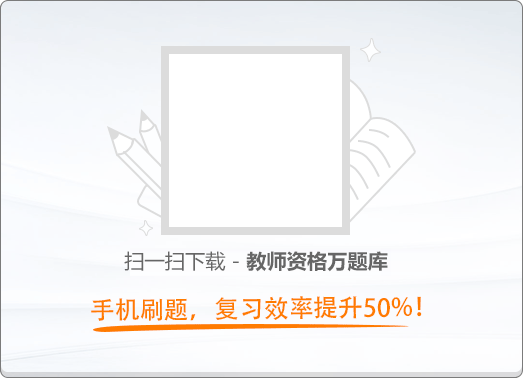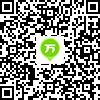120:002018年11月《幼儿综合素质》真题

1
(单项选择题)

• A.

尊重个体差异

• B.

公平对待幼儿

• C.

面向全体幼儿

• D.

引导幼儿探索

• A
• B
• C
• D

2
(单项选择题)

• A.

教师的主体作用

• B.

游戏的促进作用

• C.

幼儿的亲身体验

• D.

环境的积极影响

• A
• B
• C
• D

3
(单项选择题)

• A.

不理所有“告状”的孩子

• B.

先让孩子们安静下来再行处理问题

• C.

训所有“告状”的孩子

• D.

选取部分孩子的“告状”予以解决

• A
• B
• C
• D

4
(单项选择题)

• A.

幼儿发展知识的学习

• B.

通识性知识的学习

• C.

保教知识的学习

• D.

领域知识的学习

• A
• B
• C
• D

5
(单项选择题)

• A.

人身自由权

• B.

信仰自由权

• C.

通信自由权

• D.

教育自由权

• A
• B
• C
• D

6
(单项选择题)

• A.

18岁以下的任何人

• B.

16岁以下的任何人

• C.

10岁以下的任何人

• D.

6岁以下的任何人

• A
• B
• C
• D

7
(单项选择题)

• A.

当地人民政府

• B.

当地教育行政部门

• C.

该教育集团

• D.

该房产开发公司

• A
• B
• C
• D

8
(单项选择题)

• A.

合法，幼儿园有权管理和使用本单位经费

• B.

合法，幼儿园有按照章程自主管理的权利

• C.

不合法，侵犯了教师获取工资报酬的权利

• D.

不合法，侵犯了教师从事科学研究的自由

• A
• B
• C
• D

9
(单项选择题)

• A.

名誉权

• B.

人身自由权

• C.

生命健康权

• D.

隐私权

• A
• B
• C
• D

10
(单项选择题)

• A.

正确，幼儿园有权安排教学活动

• B.

不正确，这些内容应设在大班下学期

• C.

正确，有利于实现幼小衔接

• D.

不正确，不利于幼儿的身心发展

• A
• B
• C
• D

11
(单项选择题)

• A.

有利于全面了解幼儿健康状况

• B.

有利于选拔优秀幼儿入园

• C.

侵犯了幼儿的受教育权

• D.

侵犯了幼儿的个人隐私

• A
• B
• C
• D

12
(单项选择题)

• A.

中央人民政府教育行政部门

• B.

省级人民政府教育行政部门

• C.

市级人民政府教育行政部门

• D.

县级人民政府教育行政部门

• A
• B
• C
• D

13
(单项选择题)

• A.

未能廉洁从教

• B.

未能公平对待幼儿

• C.

未能以身作则

• D.

未能公正对待幼儿

• A
• B
• C
• D

14
(单项选择题)

• A.

正确，维护了整个活动的良好秩序

• B.

正确，保护了其他孩子的人身安全

• C.

不正确，破坏了同事间的团结协作

• D.

不正确，打击了方方的参与积极性

• A
• B
• C
• D

15
(单项选择题)

“丢手绢”的游戏结束了，张老师正准备带领小朋友们回教室，晓珺突然走到张老师面前，低声说：“老师，还没有轮到我丢手绢呢。”此时，张老师恰当的回应是（　）。

• A.

“难道还让我叫小朋友来陪你玩一次？

• B.

“待会儿我们再去玩更有趣的游戏吧！”

• C.

“小朋友们先等着，我带晓珺去玩”

• D.

“下次再玩吧，谁让你运气这么差！”

• A
• B
• C
• D

16
(单项选择题)

• A.

金老师应该注意与家长沟通的方式

• B.

生活能力培养主要由家长负责

• C.

金老师拥有批评幼儿家长的权利

• D.

生活能力培养主要由教师负责

• A
• B
• C
• D

17
(单项选择题)

• A.

• B.

• C.

小雨

• D.

毛毛雨

• A
• B
• C
• D

18
(单项选择题)

• A.

石器

• B.

陶器

• C.

瓷器

• D.

铁器

• A
• B
• C
• D

19
(单项选择题)• A.

闪电侠

• B.

蝙蝠侠

• C.

蜘蛛侠

• D.

钢铁侠

• A
• B
• C
• D

20
(单项选择题)

• A.

严文井

• B.

陈伯吹

• C.

张天翼

• D.

叶圣陶

• A
• B
• C
• D

21
(单项选择题)

• A.

《千字文》

• B.

《百家姓》

• C.

《急就章》

• D.

《山海经》

• A
• B
• C
• D

22
(单项选择题)

• A.

时间单位、时间单位

• B.

长度单位、长度单位

• C.

时间单位、长度单位

• D.

长度单位、时间单位

• A
• B
• C
• D

23
(单项选择题)

• A.

彗星

• B.

金星

• C.

流星

• D.

月亮

• A
• B
• C
• D

24
(单项选择题)

• A.

防止过量太阳之外显辐射

• B.

帮助保持地球温度

• C.

锁住地球生物所需水分

• D.

产生扼尔尼诺现象

• A
• B
• C
• D

25
(单项选择题)• A.

傣族竹楼

• B.

福建文楼

• C.

侗族鼓楼

• D.

西藏碉房

• A
• B
• C
• D

26
(单项选择题)

• A.

可以在文档中插入剪贴画

• B.

可以在文档中插入图形

• C.

可以在文档中插入文本框

• D.

可以在文档中插入配色方案

• A
• B
• C
• D

27
(单项选择题)

• A.

只能用鼠标不能用时间来控制动画

• B.

只能用时间不能用鼠标来控制动画

• C.

鼠标和时间都能够控制动画

• D.

鼠标和时间都不能控制动画

• A
• B
• C
• D

28
(单项选择题)

• A.

电冰箱—空调

• B.

坐具—双人床

• C.

消毒柜—冰柜

• D.

炊具—煤气灶

• A
• B
• C
• D

29
(单项选择题)

• A.

330

• B.

340

• C.

350

• D.

360

• A
• B
• C
• D